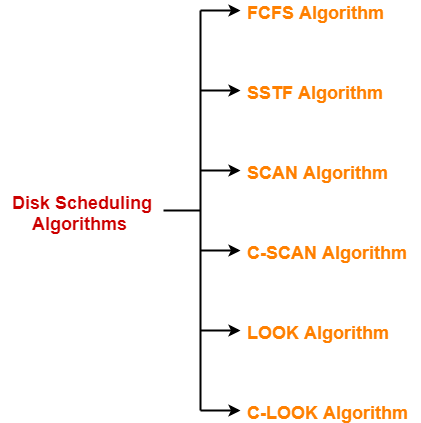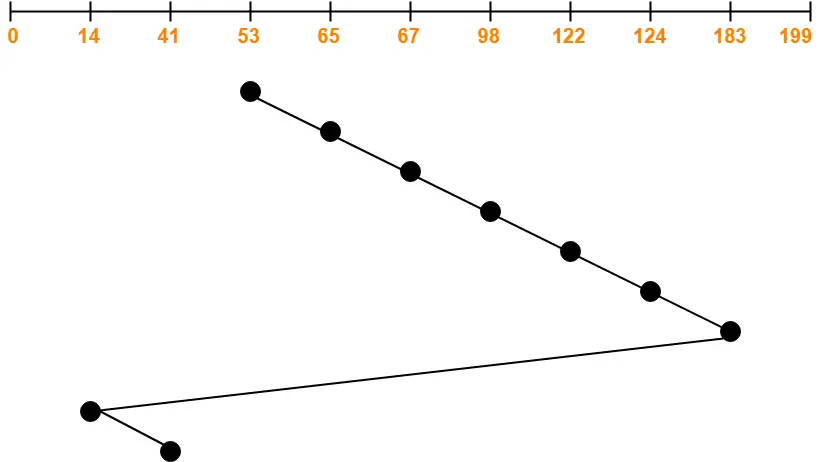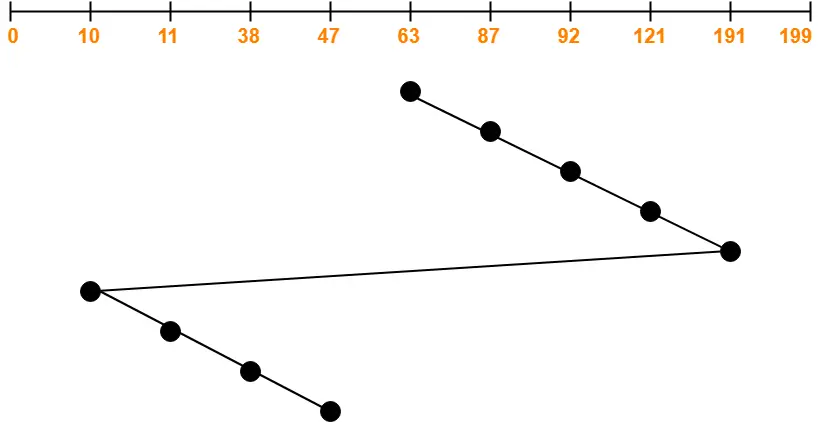# C-LOOK Algorithm | Disk Scheduling Algorithms

## Disk Scheduling Algorithms-

Before you go through this article, make sure that you have gone through the previous article on Disk Scheduling.

We have discussed-

• Disk scheduling algorithms are used to schedule multiple requests for accessing the disk.
• The purpose of disk scheduling algorithms is to reduce the total seek time.

Various disk scheduling algorithms are-## C-LOOK Disk Scheduling Algorithm-

• Circular-LOOK Algorithm is an improved version of the LOOK Algorithm.
• Head starts from the first request at one end of the disk and moves towards the last request at the other end servicing all the requests in between.
• After reaching the last request at the other end, head reverses its direction.
• It then returns to the first request at the starting end without servicing any request in between.
• The same process repeats.

Also Read- SCAN Disk Scheduling Algorithm

• It does not causes the head to move till the ends of the disk when there are no requests to be serviced.
• It reduces the waiting time for the cylinders just visited by the head.
• It provides better performance as compared to LOOK Algorithm.
• It does not lead to starvation.
• It provides low variance in response time and waiting time.

• There is an overhead of finding the end requests.

Also Read- C-SCAN Disk Scheduling Algorithm

## Problem-01:

Consider a disk queue with requests for I/O to blocks on cylinders 98, 183, 41, 122, 14, 124, 65, 67. The C-LOOK scheduling algorithm is used. The head is initially at cylinder number 53 moving towards larger cylinder numbers on its servicing pass. The cylinders are numbered from 0 to 199. The total head movement (in number of cylinders) incurred while servicing these requests is _______.

## Solution-Total head movements incurred while servicing these requests

= (65 – 53) + (67 – 65) + (98 – 67) + (122 – 98) + (124 – 122) + (183 – 124) + (183 – 14) + (41 – 14)

= 12 + 2 + 31 + 24 + 2 + 59 + 169 + 27

= 326

Alternatively,

Total head movements incurred while servicing these requests

= (183 – 53) + (183 – 14) + (41 – 14)

= 130 + 169 + 27

= 326

## Problem-02:

Consider a disk queue with requests for I/O to blocks on cylinders 47, 38, 121, 191, 87, 11, 92, 10. The C-LOOK scheduling algorithm is used. The head is initially at cylinder number 63 moving towards larger cylinder numbers on its servicing pass. The cylinders are numbered from 0 to 199. The total head movement (in number of cylinders) incurred while servicing these requests is _______.

## Solution-Total head movements incurred while servicing these requests

= (87 – 63) + (92 – 87) + (121 – 92) + (191 – 121) + (191 – 10) + (11 – 10) + (38 – 11) + (47 – 38)

= 24 + 5 + 29 + 70 + 181 + 1 + 27 + 9

= 346

Alternatively,

Total head movements incurred while servicing these requests

= (191 – 63) + (191 – 10) + (47 – 10)

= 128 + 181 + 37

= 346

To gain better understanding about C-LOOK Disk Scheduling Algorithm,

Watch this Video Lecture

Get more notes and other study material of Operating System.

Watch video lectures by visiting our YouTube channel LearnVidFun.

SummaryArticle Name
C-LOOK Algorithm | Disk Scheduling Algorithms
Description
C-LOOK Disk Scheduling Algorithm is an improved version of the LOOK Disk Scheduling Algorithm. C-LOOK Disk Scheduling Example. C-LOOK Algorithm overcomes the disadvantage of LOOK Algorithm.
Author
Publisher Name
Gate Vidyalay
Publisher Logo